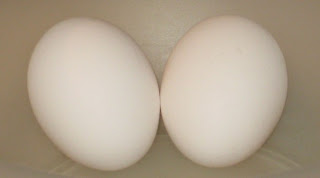Google Interview Puzzle : 2 Egg Problem...for their company. Then many companies asked this Puzzle during their

interview or written test in campus. This puzzle is famously known as 2 Egg

Problem. The puzzle is given below.

Puzzle : Two Egg Problem:

* You are given 2 eggs.
* Eggs can be very hard or very fragile means it may break if dropped from the first floor or may not even break if dropped from 100 th floor.Both eggs are identical.
* You need to figure out the highest floor of a 100-storey building an egg can be dropped without breaking.
* Now the question is how many drops you need to make. You are allowed to break 2 eggs in the process

If you are one of the people who likes to solve a puzzle before seeing the answer you must quit the blog now and come back later for checking the answer.

Now that this is a Google interview question I am taking the normal "Interview-Style" of solving a problem. Simply saying thinking aloud through the solution from worst to the best correcting the flows optimizing the solution or taking the 5-minute hard thinking acting pause to a problem, which you know already and just want to make your interviewer think that you are a challenge lover.

Let x be the answer we want, the number of drops required.

So if the first egg breaks maximum we can have x-1 drops and so we must always put the first egg from height x. So we have determined that for a given x we must drop the first ball from x height. And now if the first drop of the first egg doesn’t breaks we can have x-2 drops for the second egg if the first egg breaks in the second drop.

Taking an example, lets say 16 is my answer. That I need 16 drops to find out the answer. Lets see whether we can find out the height in 16 drops. First we drop from height 16,and if it breaks we try all floors from 1 to 15.If the egg don’t break then we have left 15 drops, so we will drop it from 16+15+1 =32nd floor. The reason being if it breaks at 32nd floor we can try all the floors from 17 to 31 in 14 drops (total of 16 drops). Now if it did not break then we have left 13 drops. and we can figure out whether we can find out whether we can figure out the floor in 16 drops.

Lets take the case with 16 as the answer

1 + 15 16 if breaks at 16 checks from 1 to 15 in 15 drops
1 + 14 31 if breaks at 31 checks from 17 to 30 in 14 drops
1 + 13 45 .....
1 + 12 58
1 + 11 70
1 + 10 81
1 + 9 91
1 + 8 100 We can easily do in the end as we have enough drops to accomplish the task

Now finding out the optimal one we can see that we could have done it in either 15 or 14 drops only but how can we find the optimal one. From the above table we can see that the optimal one will be needing 0 linear trials in the last step.

So we could write it as

(1+p) + (1+(p-1))+ (1+(p-2)) + .........+ (1+0) >= 100.

Let 1+p=q which is the answer we are looking for

q (q+1)/2 >=100

Solving for 100 you get q=14.